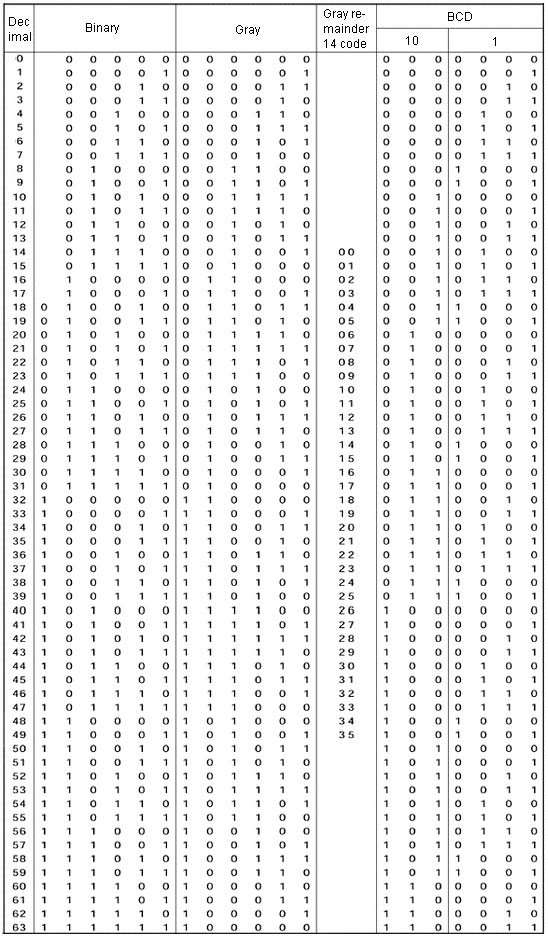What is "Absolute code"?

1. Binary Code

A pure binary code, expressed in the format 2n.

Multiple bits may change when an address changes.

2. Gray Code

A code in which only one bit changes when an address changes.

The code plate of the Rotary Encoder uses gray code.

3. Remainder Gray Code

This code is used when expressing resolutions with gray code that are not 2n, such as 36, 360, and 720. The nature of gray code is such that when the most significant bit of the code changes from 0 to 1 and the same size of area is used for both the larger value and the smaller value of objects, the signal only changes by 1 bit within this range when changing from the end to the beginning of a code.

This enables any resolution that is an even number to be set with gray code. In this case, the code does not begin from 0, but from an intermediate code, and thus when actually using a code it must first be shifted so that it starts from 0.

The example in the code table shows 36 divisions. For the change from address 31 to 32, the code extends from address 14 to 49 when 18 addresses each are taken for the objects. When changing from address 49 to 14, only one bit changes, and we can see that the characteristic of gray code is preserved. By shifting the code 14 addresses, it can be converted to a code that starts from address 0.

4. BCD

Binary Coded Decimal Code.

Each digit of a decimal number is expressed using a binary value.

Absolute code table is as follows.